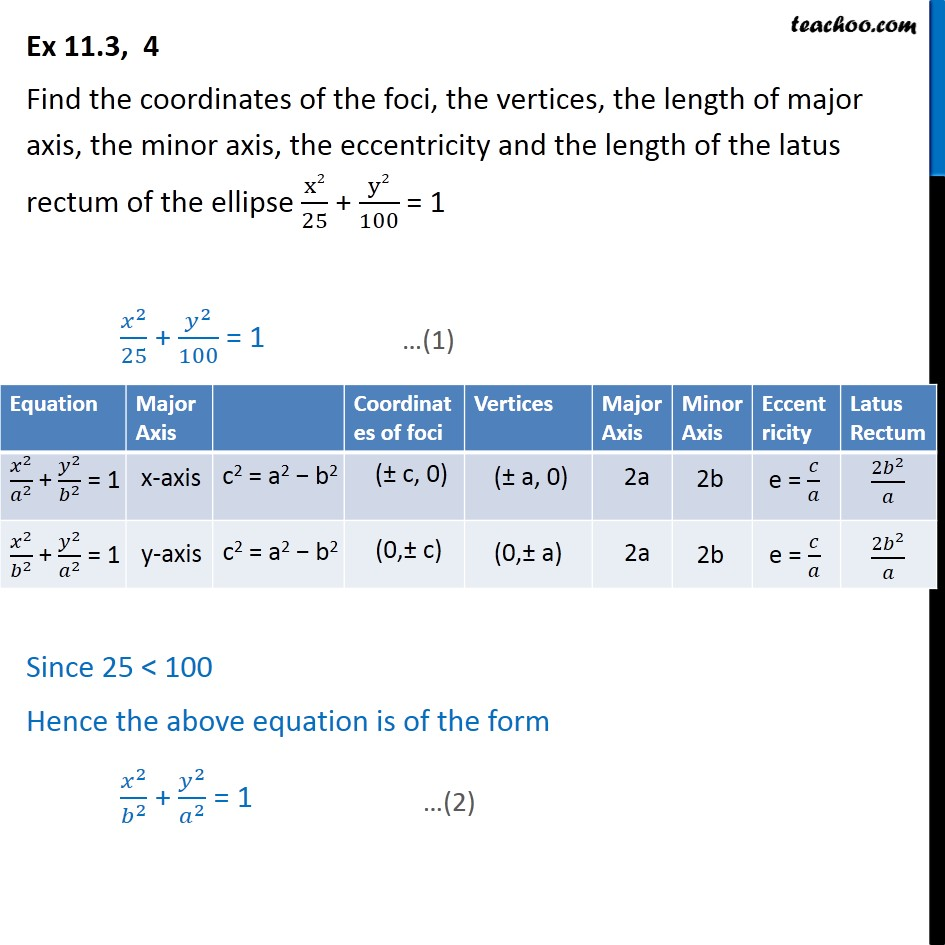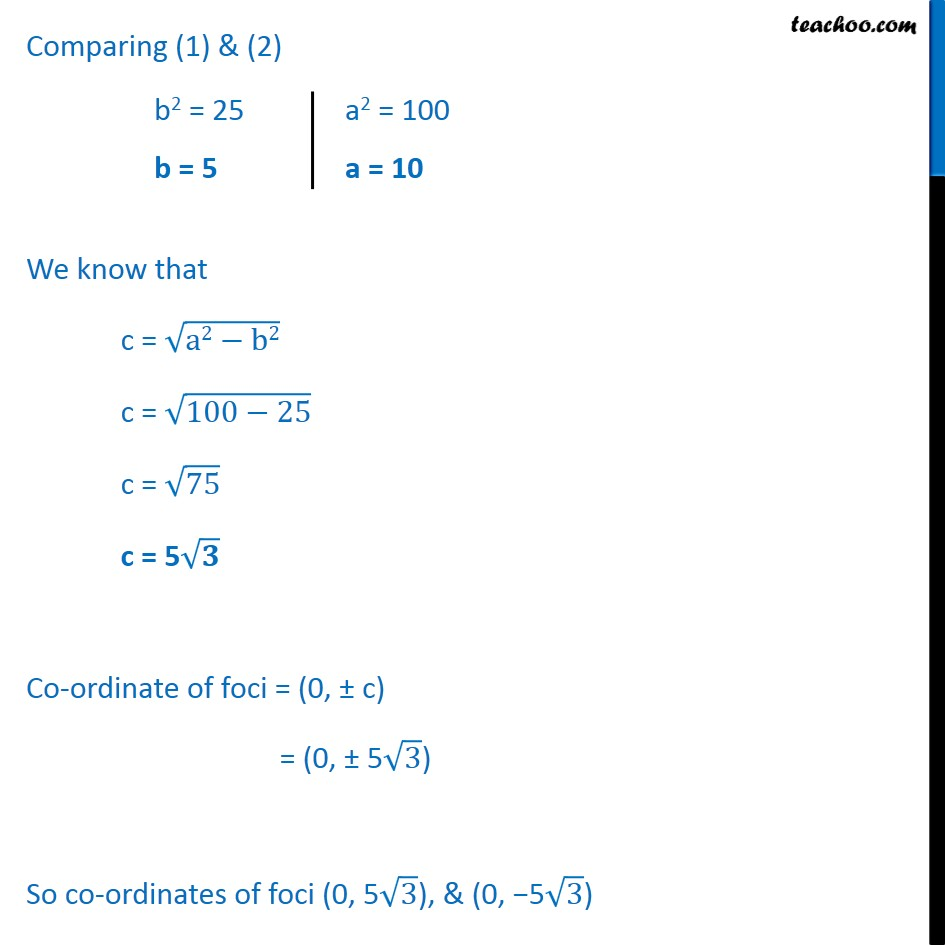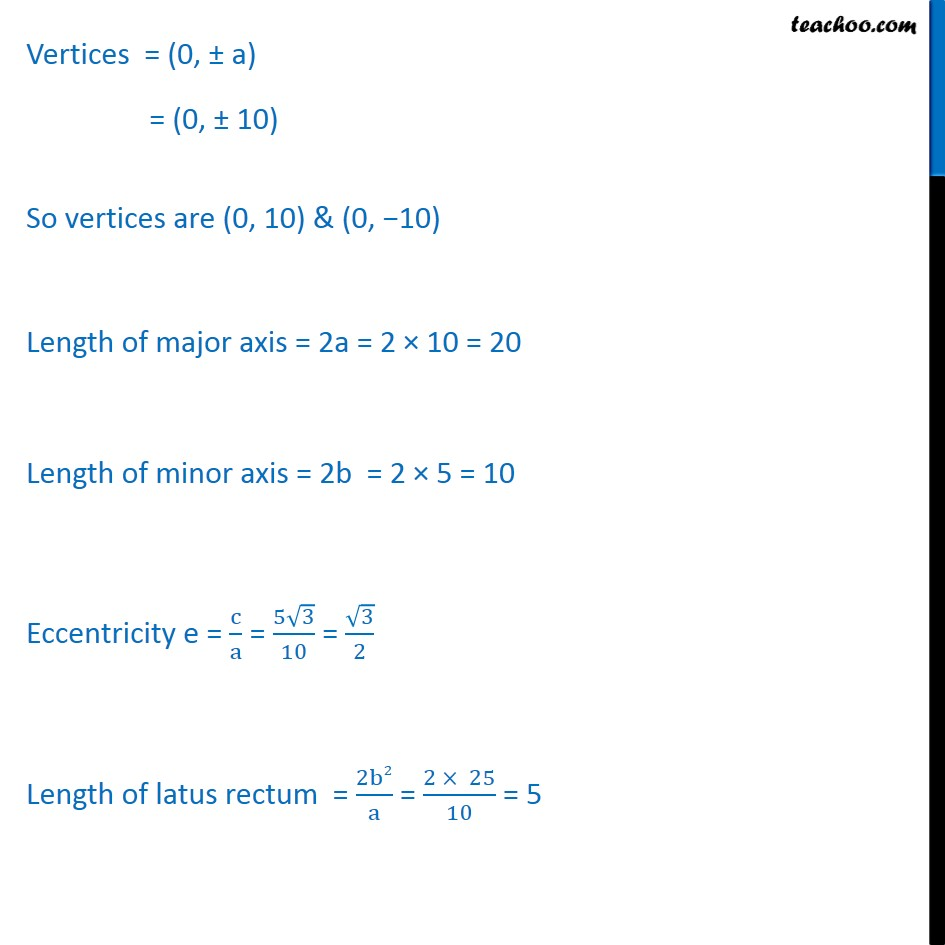Ellipse - Defination

Chapter 10 Class 11 Conic Sections
Concept wiseLearn in your speed, with individual attention - Teachoo Maths 1-on-1 Class

### Transcript

Ex 10.3, 4 Find the coordinates of the foci, the vertices, the length of major axis, the minor axis, the eccentricity and the length of the latus rectum of the ellipse x2 25 + y2 100 = 1 2 25 + 2 100 = 1 Since 25 < 100 Hence the above equation is of the form 2 2 + 2 2 = 1 Comparing (1) & (2) We know that c = a2 b2 c = 100 25 c = 75 c = 5 Co-ordinate of foci = (0, c) = (0, 5 3 ) So co-ordinates of foci (0, 5 3 ), & (0, 5 3 ) Vertices = (0, a) = (0, 10) So vertices are (0, 10) & (0, 10) Length of major axis = 2a = 2 10 = 20 Length of minor axis = 2b = 2 5 = 10 Eccentricity e = c a = 5 3 10 = 3 2 Length of latus rectum = 2b2 a = 2 25 10 = 5